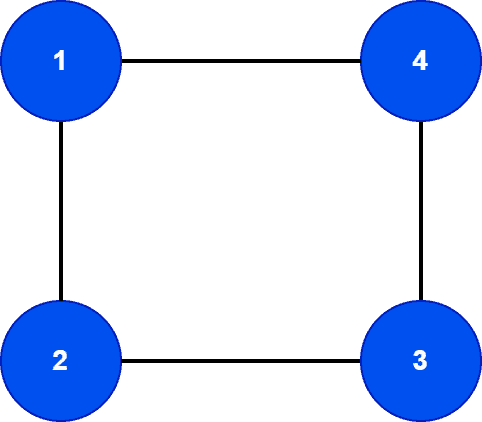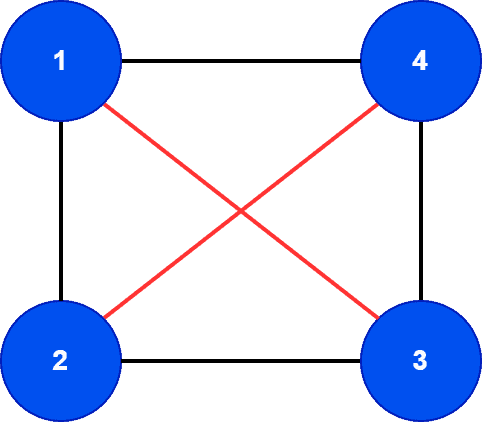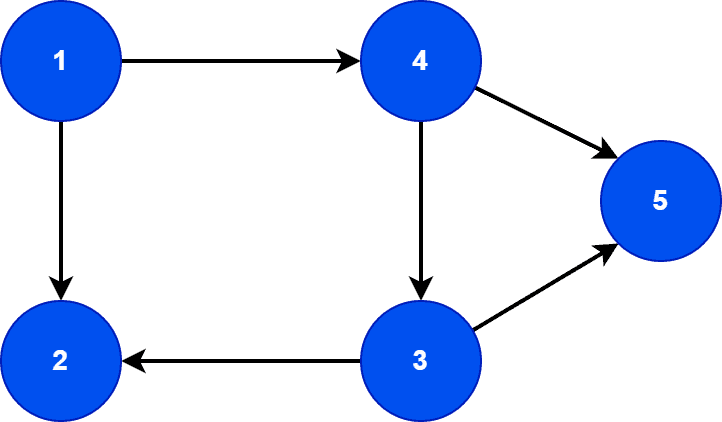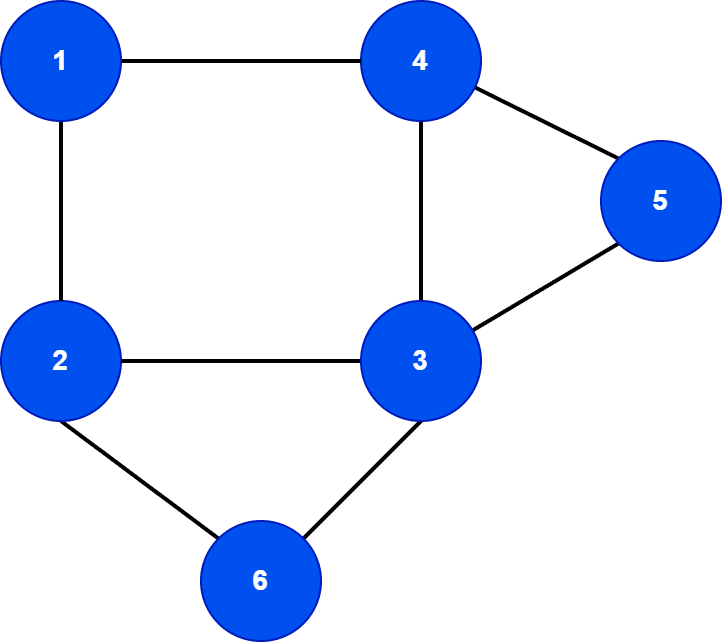## 1. Overview

In this tutorial, we’ll discuss the density of a graph. We’ll present how to calculate the density of different graphs with examples.

## 2. What Is Density?

Graph density represents the ratio between the edges present in a graph and the maximum number of edges that the graph can contain. Conceptually, it provides an idea of how dense a graph is in terms of edge connectivity.

It’s particularly useful when we have a vast network and want to add new edges to the network. Moreover, graph density gives us an idea of how many edges we can still add to the network.

Now, before deriving the formula for graph density, let’s talk about how to calculate the maximum number of edges in a simple directed and undirected graph. Let’s look at a simple undirected graph:Here, the graphcontainsnodes andedges. We’re interested in knowing the maximum number of edgescan contain. Can we still add edges? Let’s see:We addedmore edges to the graph. Therefore, the graph now containsnodes andedges. We can’t add any more edges to it. Now, we want to derive a standard formula to calculate the maximum number of edges in a simple undirected graph:Let’s check the formula for:Similarly, we can replace each undirected edge with two bidirectional edges in a directed graph. Hence, the maximum number of edges can be calculated using a similar formula:We can now define the graph density formula. The edges present in a graphare divided by the maximum number of edges that the graph can contain. Let’s see the formula for a simple undirected graph:Similarly, we can rewrite the previous density formula for a directed graph:## 3. Properties

For a complete directed or undirected graph, the density is always. Therefore, if we recollect the definition, we can easily verify this property. The density is the ratio of edges present in a graph divided by the maximum possible edges. In the case of a complete directed or undirected graph, it already has the maximum number of edges, and we can’t add any more edges to it. Hence, the density will be. Additionally, it also indicates the graph is fully dense.

A graph with all isolated vertices has a density of. Moreover, it denotes there are no edges in the graph, and the number of edges that can be added to the graph is equal to the maximum number of edges.

## 4. Example

In this section, we’ll calculate the density for a directed and an undirected graph. Let’s take a directed graphfirst:The directed graphhasvertices andedges. But in order to calculate density, first, we need to calculate the maximum number of edges possible in:Finally, we divide the number of edge present inwith the maximum number of edges in order to calculate density:Similarly, let’s take an undirected graph:The undirected graphhasvertices andedges. First, we’ll calculate the maximum number of edges for:Finally, we divide the number of edges present inwith the maximum number of edges in order to calculate density:## 5. Conclusion

In this tutorial, we discussed graph density in detail. We first derived the graph density formula. We also explained the calculation using a directed and an undirected graph.